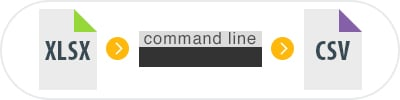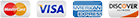# Convert XLSX to CSV Via Command Line

Excel is a wonderful piece of software in general but cracks quickly begin to show when you need to convert Excel-authored content to other formats such as CSV in bulk. It is a common requirement though in all sorts of commercial settings and one that is really better handled by a dedicated solution - ideally one that can run quietly on a server in the background while you concentrate on your work.Total Excel Converter X is our flagship server XLSX CSV converter solution. It incorporates all of the power of the desktop version of the software with the ability to run it directly on a server or even integrate it into your own web-based applications through its support for ActiveX.

The advantages of having a server based XLSX converter to hand are numerous and the software ships with a number of specific features that make conversion to CSV particularly easy:

• You can convert several XLSX files into one CSV.
• Use the built-in renamer options to add elements such as worksheet names or counters to your file names.
• Add barcodes or logos to the header or footer of pages.
• Choose from a range of standard delimiters or define your own when converting.
• Use the built-in fit-to-page functionality to avoid having to step through individual files post-conversion.

Total Excel Converter X runs quietly in the background on your server without pestering you with interrupting messages or forcing you to step through a GUI. It can also be called directly from the terminal and the ability to convert XLSX to CSV via command line is a huge productivity boost in all manner of scenarios.

Total Excel Converter X is the product of many years of iterative improvement and refinement through extensive user testing. It is a heavyweight, server based tool available at a fraction of the price of competing solutions and orders of magnitude cheaper than developing similar functionality in-house.

With thousands of happy customers worldwide, we are confident that our software will match your conversion requirements. That is why we urge you to download a free trial today and discover its full range of features for yourself before purchasing!## Examples of Total Excel ConverterX

### Convert excel files With TotalExcelConverterX and .NET

```string src="C:\\test\\Source.xlsx";
string dest="C:\\test\\Dest.PDF";

ExcelConverterX Cnv = new ExcelConverterX();
Cnv.Convert(src, dest, "-c PDF -log c:\\test\\Excel.log");

MessageBox.Show("Convert complete!");
```

### Convert Excel Files On Web Servers With Total Excel ConverterX

```dim C
Set C=CreateObject("ExcelConverter.ExcelConverterX")
C.Convert "c:\test\source.xlsx", "c:\test\dest.pdf", "-cPDF -log c:\test\Excel.log"
Response.Write C.ErrorMessage
set C = nothing
```
Example2 ASP: directly stream the resulting PDF
```dim C
Set C=CreateObject("ExcelConverter.ExcelConverterX")
Response.Clear
Response.BinaryWrite c.ConvertToStream("C:\www\ASP\Source.xlsx", "C:\www\ASP", "-cpdf  -log c:\html.log")
set C = nothing
```

### Convert Excel Spreadsheets by PHP With Total Excel ConverterX

```\$src="C:\\test\\test.XLS";
\$dest="C:\\test\\test.CSV";
\$c= new COM("ExcelConverter.ExcelConverterX");
\$c->convert(\$src,\$dest, "-c csv  -log c:\\test\\xls.log");
if (file_exists(\$dest)) echo "OK"; else echo "fail:".\$c->ErrorMessage;
```

### Convert Excel Spreadsheets By Ruby With Total Excel ConverterX

```require 'win32ole'
c = WIN32OLE.new('ExcelConverter.ExcelConverterX')

src="C:\\test\\test.xlsx";
dest="C:\\test\\test.pdf";

c.convert(src,dest, "-c PDF -log c:\\test\\Excel.log");

if not File.exist?(dest)
puts c.ErrorMessage
end

```

### Convert Excel Spreadsheets by Python With Total Excel ConverterX

```import win32com.client
import os.path

c = win32com.client.Dispatch("ExcelConverter.ExcelConverterX")

src="C:\\test\\test.xlsx";
dest="C:\\test\\test.pdf";

c.convert(src, dest, "-c PDF -log c:\\test\\Excel.log");

if not os.path.exists(file_path):
print(c.ErrorMessage)
```

### Convert Excel files With Pascal and Total Excel ConverterX

```uses Dialogs, Vcl.OleAuto;

var
c: OleVariant;
begin
c:=CreateOleObject('ExcelConverter.ExcelConverterX');
C.Convert('c:\test\source.xlsx', 'c:\test\dest.pdf', '-cPDF -log c:\test\Excel.log');
IF c.ErrorMessage<> Then
ShowMessage(c.ErrorMessage);
end;
```

### Convert Excel and ODS Files On Web Servers With Total Excel ConverterX

```var c = new ActiveXObject("ExcelConverter.ExcelConverterX");
c.Convert("C:\\test\\source.xlsx", "C:\\test\\dest.pdf", "-c PDF");
if (c.ErrorMessage!="")
```

### Convert Excel Spreadsheets by Perl With Total Excel ConverterX

```use Win32::OLE;

my \$src="C:\\test\\test.XLS";
my \$dest="C:\\test\\test.CSV";

my \$c = CreateObject Win32::OLE 'ExcelConverter.ExcelConverterX';
\$c->convert(\$src,\$dest, "-c csv  -log c:\\test\\xls.log");
print \$c->ErrorMessage if -e \$dest;
```

### Latest News

• New Total PDF Printer has been released. Now you can print a folder of PDF files and then move the files to a different folder (-msuccess).
• We have fixed an issue in Coolutils Converter where some messages in HTML were not showing correctly.
• New Total HTML Converter has got an update with a few important bug fixes (large radius, paged layout).
• New Total Mail Converter Pro offers enhanced option to fit extra wide tables from emails to PDF files.
• New PDF Combine 7.0 is live. New options for creating combined PDF scans, e-books and songbooks.
• New Total Excel Converter 6.0 is live. Convert XLS with with charts.